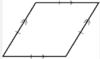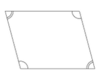# Always, Sometimes, Never

Alignments to Content Standards: 5.G.B.3

Decide whether each of these statements is always, sometimes, or never true.  If it is sometimes true, draw and describe a figure for which the statement is true and another figure for which the statement is not true.

1. A rhombus is a square
2. A triangle is a parallelogram
3. A square is a parallelogram
4. A square is a rhombus
5. A parallelogram is a rectangle
6. A trapezoid is a quadrilateral

## IM Commentary

The purpose of this task is to have students reason about different kinds of shapes based on their defining attributes and to understand the relationship between different categories of shapes that share some defining attributes. In cases when the list of defining attributes for the first shape is a subset of the defining attributes of the second shape, then the statements will always be true. In cases when the list of defining attributes for the second shape is a subset of the defining attributes of the first shape, then the statements will sometimes be true.

When this task is used in instruction, teachers should be prioritizing the Standard for Mathematical Practice 6: Attend to Precision. Students should base their reasoning by referring to side length, side relationships, and angle measures.

## Solution

1. A rhombus is a square.

This is sometimes true.  It is true when a rhombus has 4 right angles.  It is not true when a rhombus does not have any right angles.

Here is an example when a rhombus is a square:Here is an example when a rhombus is not a square:2. A triangle is a parallelogram.

This is never true.  A triangle is a three-sided figure.  A parallelogram is a four-sided figure with two sets of parallel sides.

3. A square is a parallelogram.

This is always true.  Squares are quadrilaterals with 4 congruent sides and 4 right angles, and they also have two sets of parallel sides. Parallelograms are quadrilaterals with two sets of parallel sides. Since squares must be quadrilaterals with two sets of parallel sides, then all squares are parallelograms.

4. A square is a rhombus

This is always true.  Squares are quadrilaterals with 4 congruent sides.  Since rhombuses are quadrilaterals with 4 congruent sides, squares are by definition also rhombuses.

5. A parallelogram is a rectangle.

This is sometimes true.  It is true when the parallelogram has 4 right angles.  It is not true when a parallelogram has no right angles.

Here is an example when a parallelogram is a rectangle:Here is an example when a parallelogram is not a rectangle:6. A trapezoid is a quadrilateral.

This is always true.  Trapezoids must have 4 sides, so they must always be quadrilaterals.